### Selection and evolution in B cells

Most slides from Sarah Cobey ; some from Erick

### B cells determine antibody diversity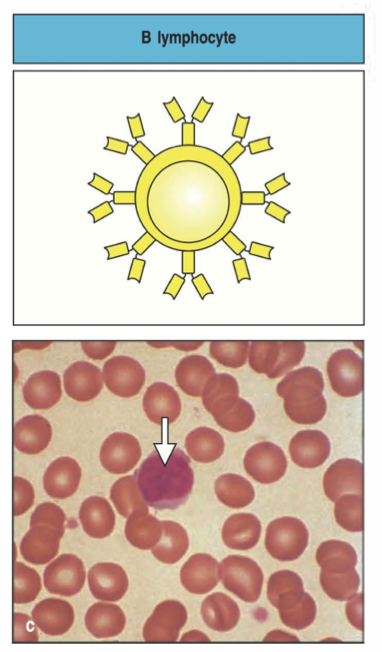### Structure of a B cell receptor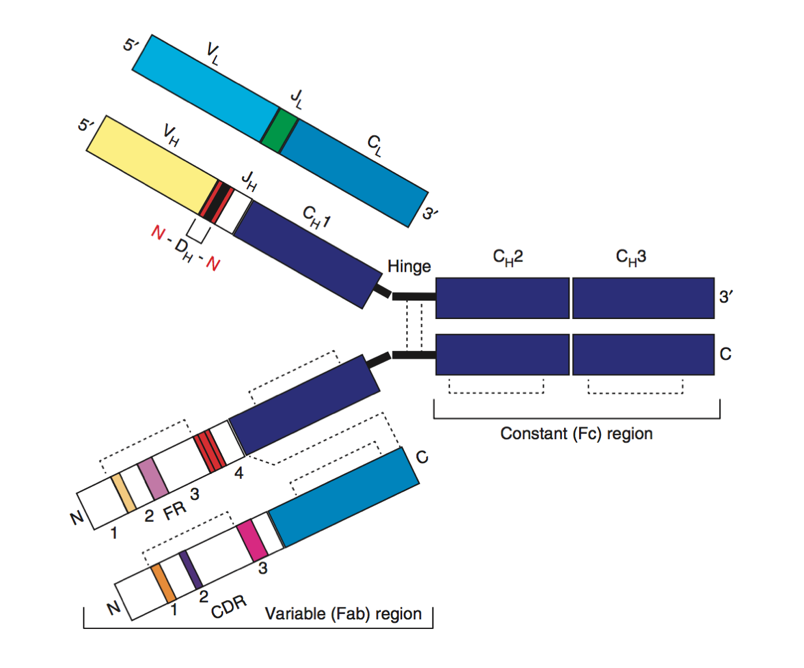### Origins of B cell receptor diversity

VDJ recombination

Affinity maturation

### VDJ loci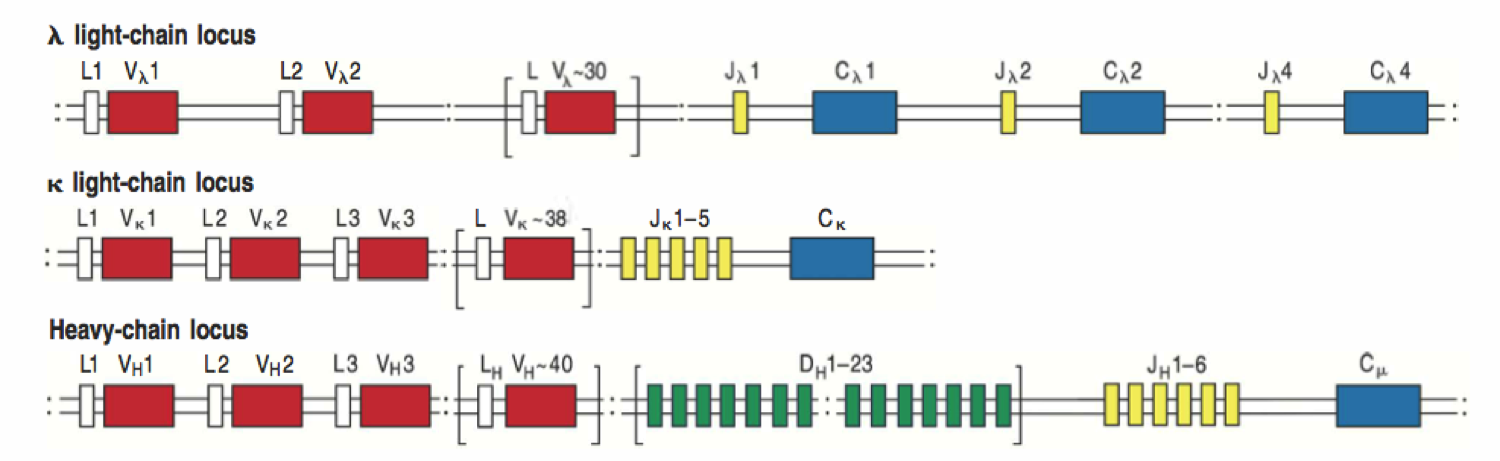### VDJ recombination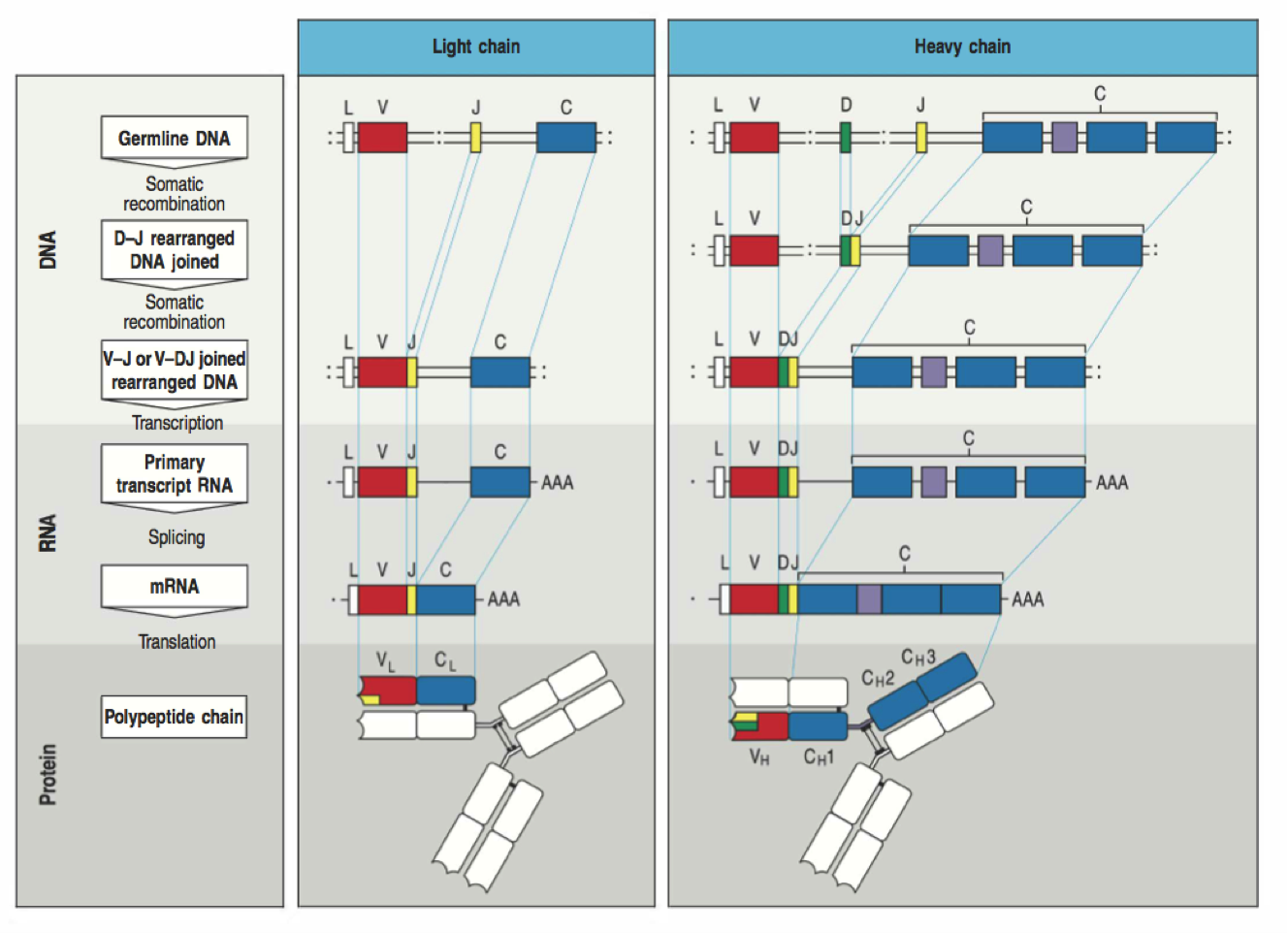### Selection on naive repertoire

Stability

Autoreactivity### Somatic hypermutation is AID + repair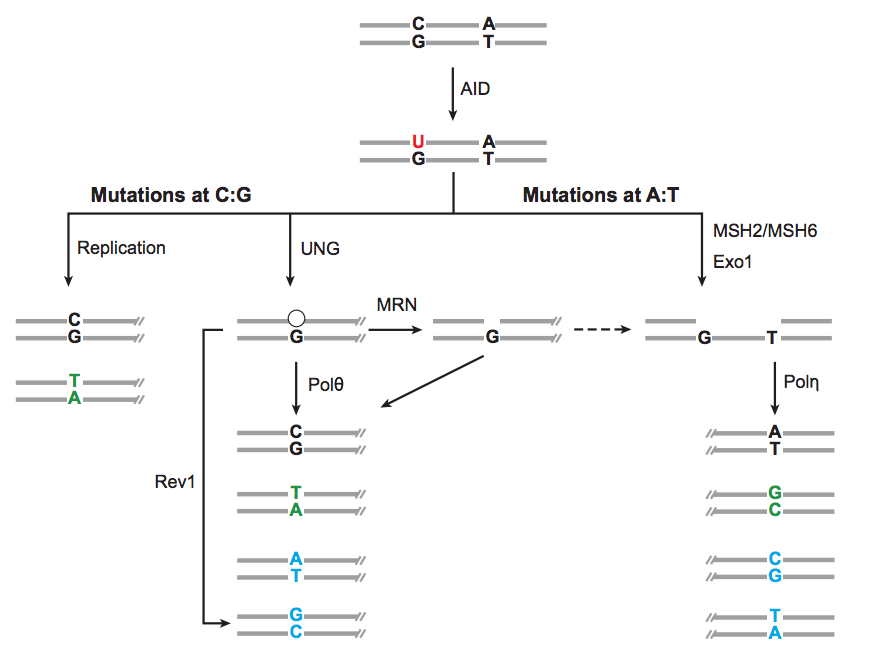### Mutation rate varies over BCR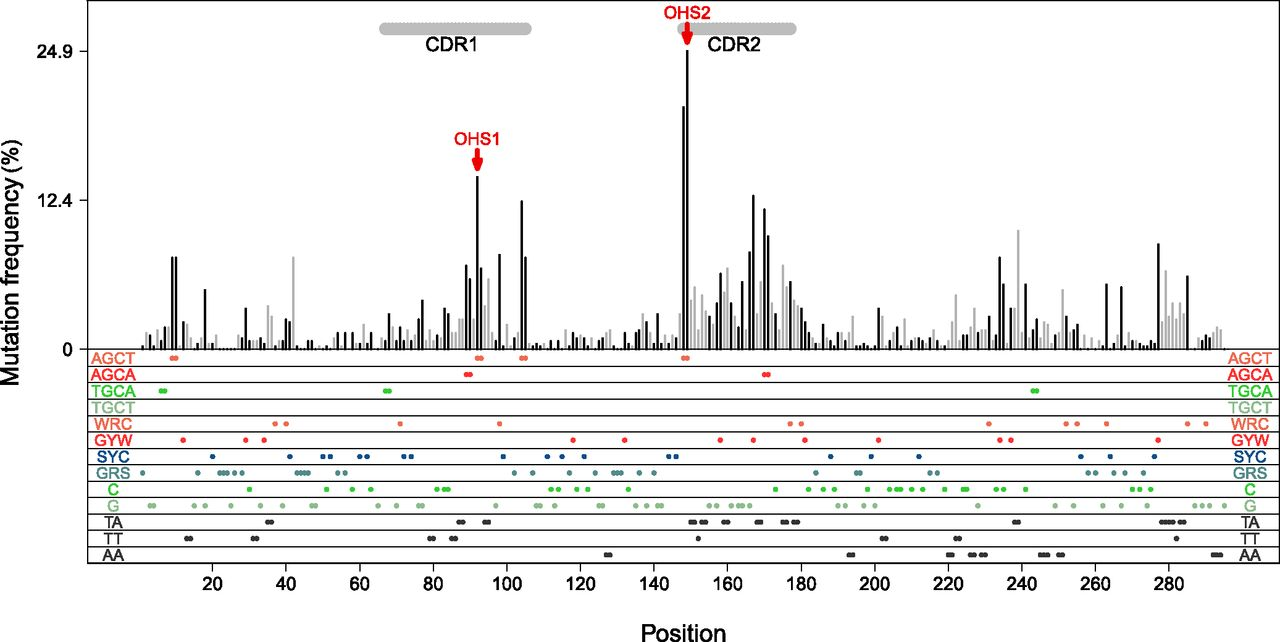### The mutations have peculiar biases### The mutations have peculiar biases### One would like to quantify these biases### Phylogenetics is not straightforward### GC B cells evolve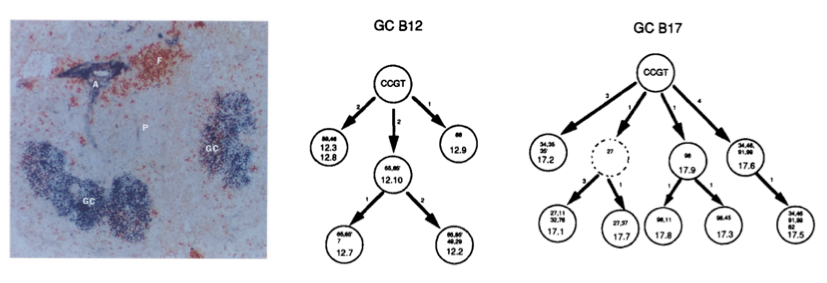### Permissive selection vs complex antigens### Memory cells can affinity mature### Antibody isotypes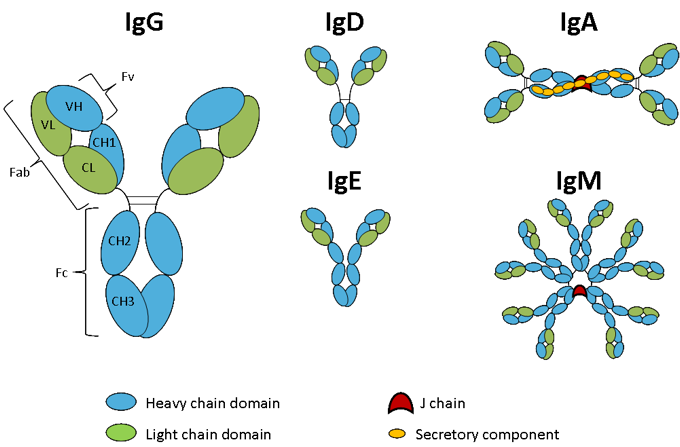### Isotype switching### Clones can be long-lived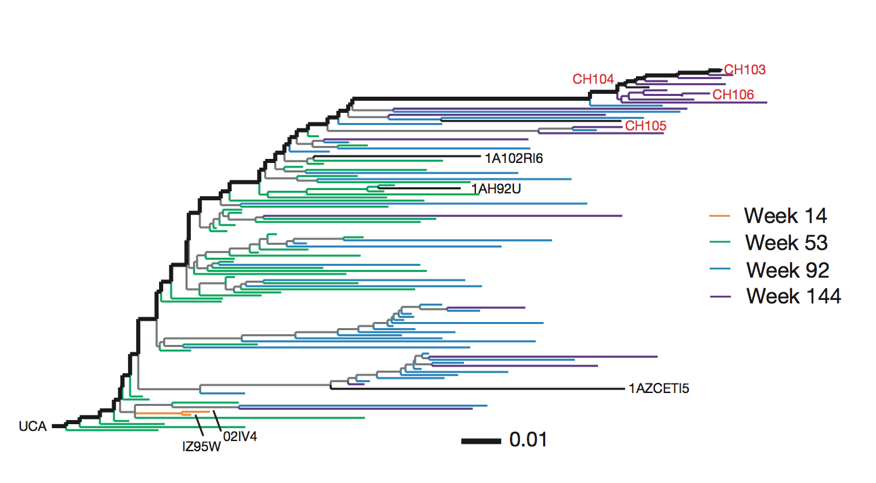### Broadly neutralizing antibodies are often highly diverged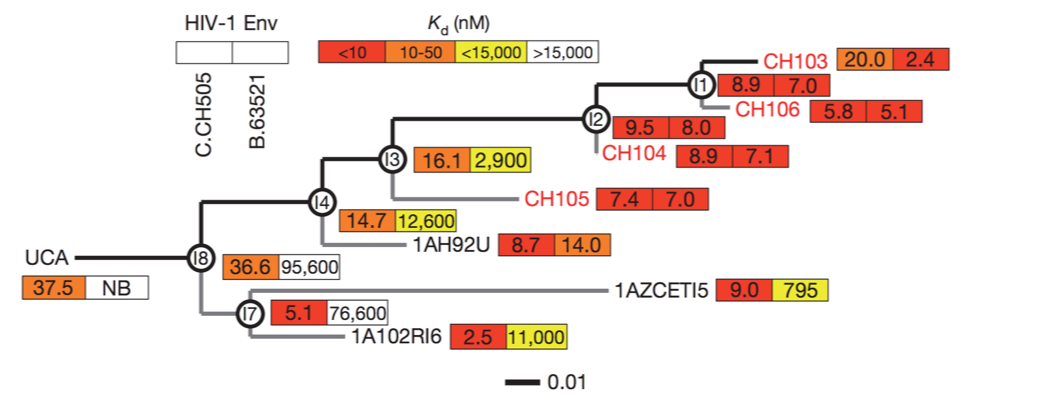### Broadly neutralizing antibodies to flu elusive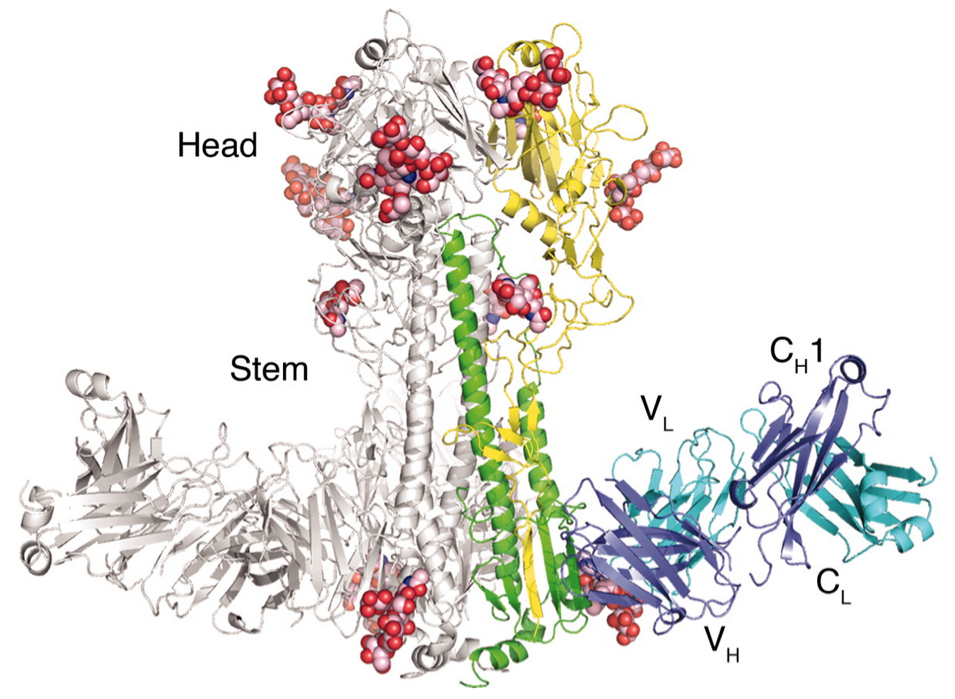Why do some people develop broadly neutralizing antibodies?

Can we induce them in everyone?

Will they dominate?

### "Restricted" responses to epitopes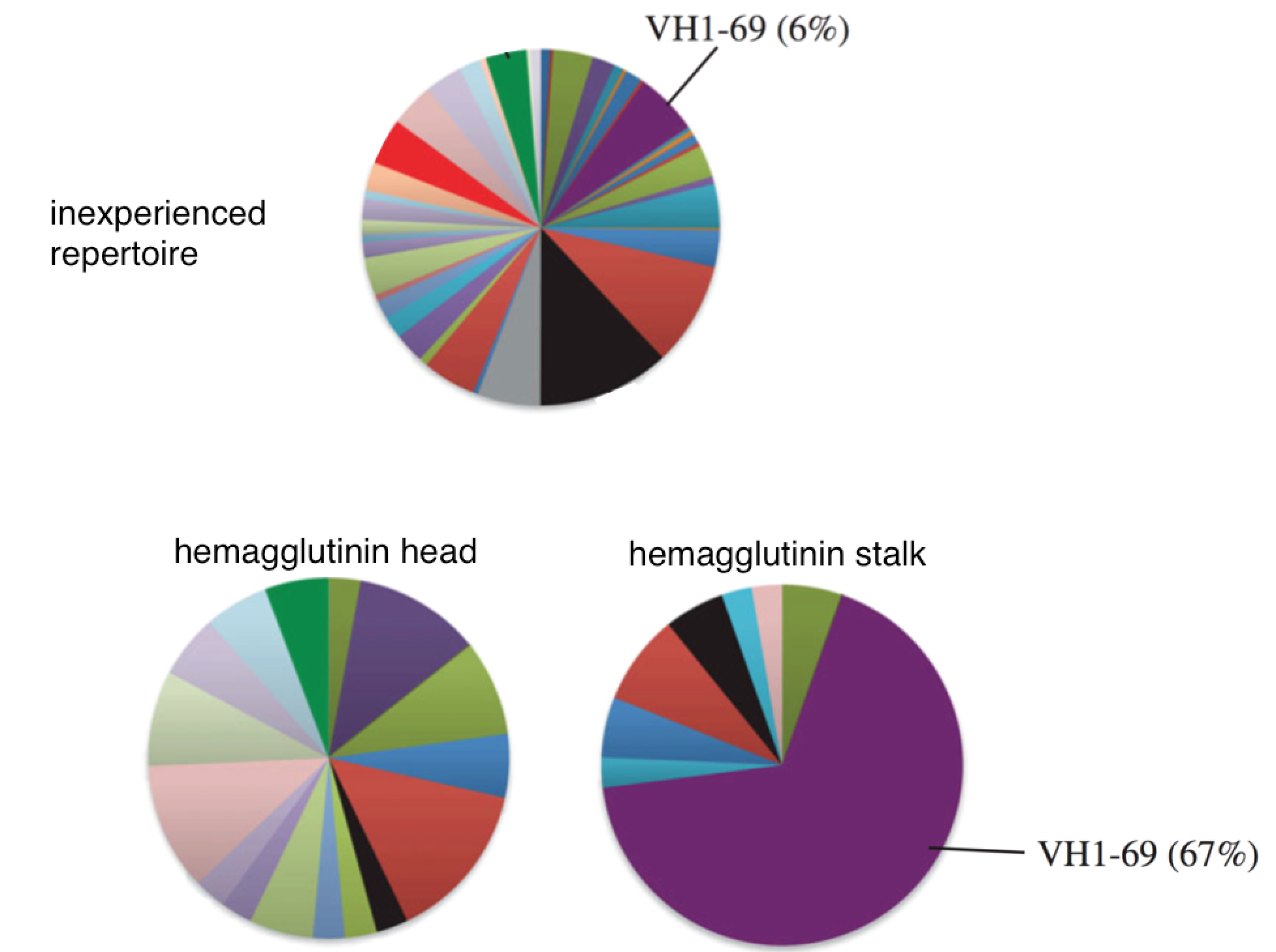### Genotype affects response to H5N1 vaccination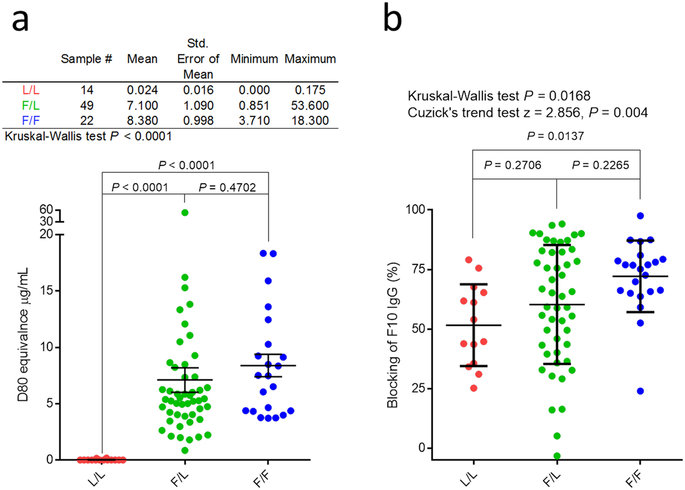### Allele copy number affects clone frequency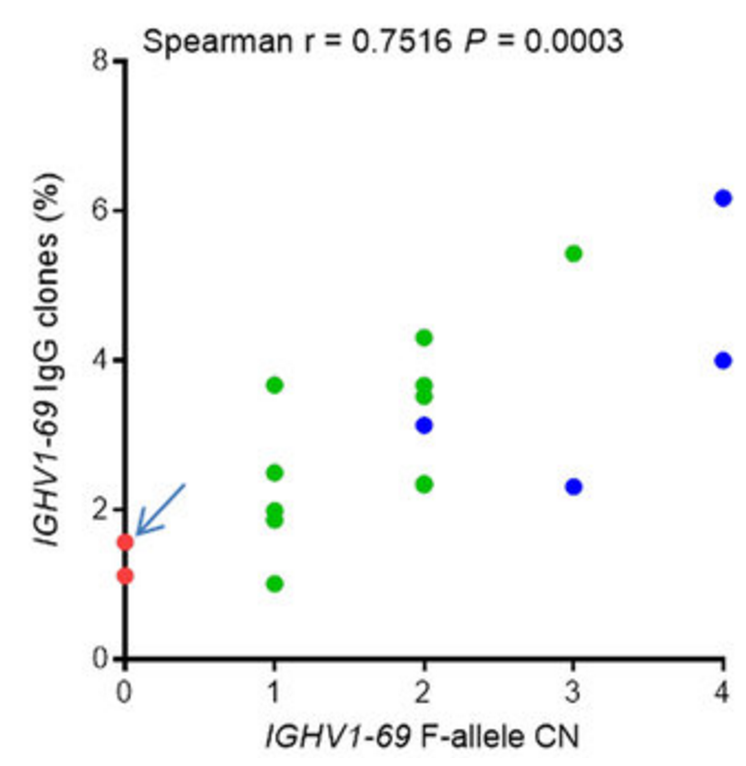### Ethnic differences in copy number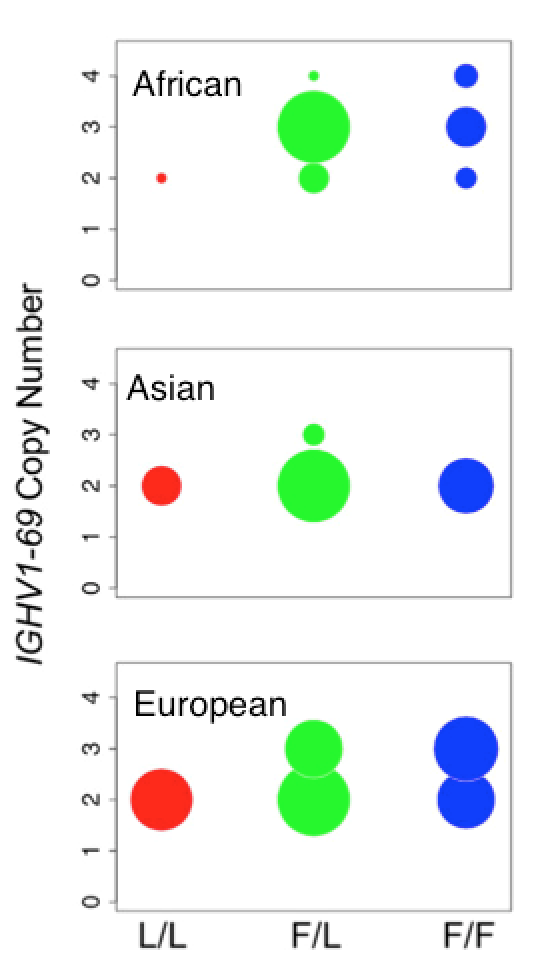# How can we learn using probabilistic models?

We don’t need lots of little techniques…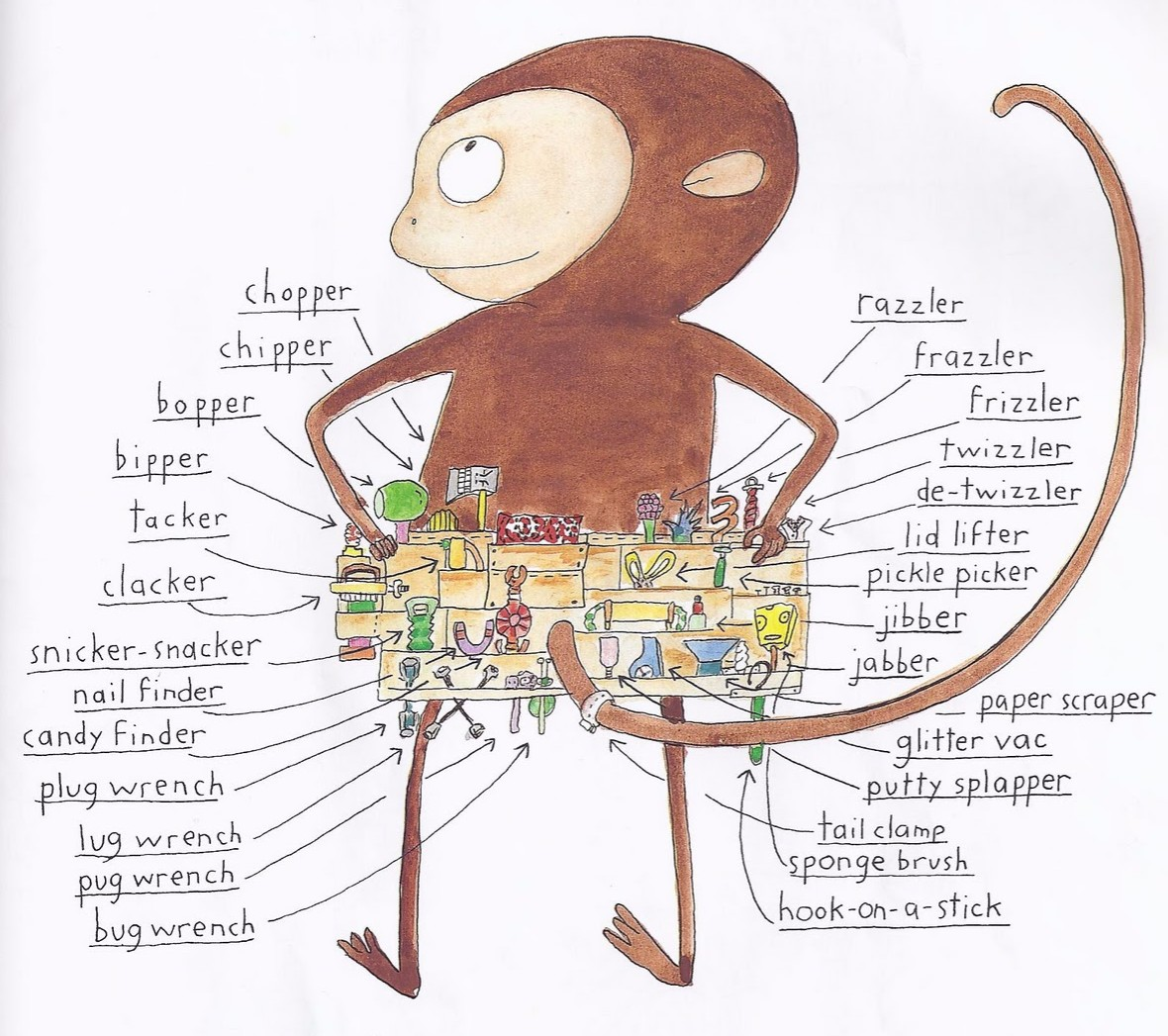we only need models and one principle: likelihood.

### We can sequence BCRs in high throughput### How do we learn from BCR sequences?1. Develop probabilistic model of biological process and how data is generated.
2. Find parameter choices that maximize the likelihood of generating the observed data.

When a field of bioinformatics is mature, it becomes statistics.
E.g. Maximum-likelihood phylogenetics, HMMER, DESeq2, etc.

### VDJ rearrangement### HMM intro: dishonest casino### HMM intro: dishonest casino### HMM intro: dishonest casino### HMM intro: dishonest casino1. What is the likelihood of the data under this path (write equation)
2. If $$p$$ is close to 0.5, what is an alternate path that will have a higher likelihood? What is this likelihood?Biased die roll $$\leftrightarrow$$ read base (under mutation)
Switching dice $$\leftrightarrow$$ changing between V, D, J genesVDJ annotation problem: from where did each nucleotide come?

A: Take maximum-likelihood HMM path.

### What are probabilities?### Distributions are reproducibly weird!### Find clonal families### Find clonal familiesDid two sequences come from the same VDJ recombination?

### Say we are given two sequences### Double roll $$\leftrightarrow$$ Pair HMM### Double roll $$\leftrightarrow$$ Pair HMMPick double roll hypothesis if it has higher likelihood of generating data.(But we only know the sequences, not the annotations!)### Do two sequences come from a single rearrangement event?

Probability of generating observed sequence $$x$$ from HMM:

$\mathbb P(x) = \sum_{\text{paths}\ \sigma} \mathbb P(x;\sigma),$

Probability of generating two sequences $$x$$ and $$y$$ from the same path through the HMM (i.e. from the same rearrangement event):

$\mathbb P(x,y) = \sum_{\text{paths}\ \sigma} \mathbb P(x,y;\sigma),$

$\text{Calculate: } \frac{\mathbb P(x, y)}{\mathbb P(x) \mathbb P(y)} = \frac{\mathbb P(\text{single rearrangement})}{\mathbb P(\text{independent rearrangements})}$

### Do sets of sequences come from a single rearrangement event?

$\frac{\mathbb P(A \cup B)}{\mathbb P(A) \mathbb P(B)} = \frac{\mathbb P(A \cup B \ | \ \text{single rearrangement})}{\mathbb P(A,B \ | \ \text{independent rearrangements})}$

Use this for agglomerative clustering; stop when the ratio < 1.• Munshaw, S., & Kepler, T. B. (2010). SoDA2: a Hidden Markov Model approach for identification of immunoglobulin rearrangements. Bioinformatics.
• Murugan, Mora, Walczak, & Callan (2012). Statistical inference of the generation probability of T-cell receptors from sequence repertoires. PNAS.
• Ralph & M. (2016). Consistency of VDJ Rearrangement and Substitution Parameters Enables Accurate B Cell Receptor Sequence Annotation. PLOS Computational Biology.
• Ralph & M. (2016). Likelihood-based inference of B-cell clonal families.PLOS Computational Biology.
• Elhanati, Sethna, Marcou, Callan, Mora, \& Walczak (2015). Inferring processes underlying B-cell repertoire diversity. Philosophical Transactions of the Royal Society of London.
• Elhanati, Marcou, Mora, & Walczak (2016). repgenHMM: a dynamic programming tool to infer the rules of immune receptor generation from sequence data. Bioinformatics.